Courses

# Test: Chemical Kinetics 1 - From Past 28 Years Questions

## 17 Questions MCQ Test Chemistry Class 12 | Test: Chemical Kinetics 1 - From Past 28 Years Questions

Description
This mock test of Test: Chemical Kinetics 1 - From Past 28 Years Questions for NEET helps you for every NEET entrance exam. This contains 17 Multiple Choice Questions for NEET Test: Chemical Kinetics 1 - From Past 28 Years Questions (mcq) to study with solutions a complete question bank. The solved questions answers in this Test: Chemical Kinetics 1 - From Past 28 Years Questions quiz give you a good mix of easy questions and tough questions. NEET students definitely take this Test: Chemical Kinetics 1 - From Past 28 Years Questions exercise for a better result in the exam. You can find other Test: Chemical Kinetics 1 - From Past 28 Years Questions extra questions, long questions & short questions for NEET on EduRev as well by searching above.
QUESTION: 1

### Half life period of a first-order reaction is 1386 seconds. The specific rate constant of the reaction is: 

Solution:

For a first order reactionQUESTION: 2

### For the reaction A + B →  products, it is observed that:  (1) On doubling the initial concentration of A only, the rate of reaction is also doubled and (2) On doubling the initial concentrations of both A and B, there is a change by a factor of 8 in the rate of the reaction. The rate of this reaction is given by:

Solution:

When concentration A is doubled, rate is doubled. Hence order with respect to A is one.
When concentrations of both A and B are doubled, rate increases by 8 times hence order with respect to B is 2.
∴   rate = k [A]1 [B]2
Total order = 1 + 2 = 3

QUESTION: 3

### For the reaction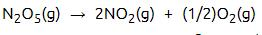the value of rate of disappearance of N2O5 is given as  6.25 × 10–3 mol L–1s–1. The rate of formation of NO2 and O2 is given respectively as : 

Solution: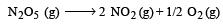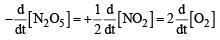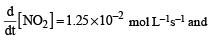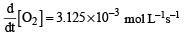QUESTION: 4

For an endothermic reaction, energy of activation is Ea and enthalpy of reaction of ΔH (both of these in kJ/mol). Minimum value of Ea will be. 

Solution: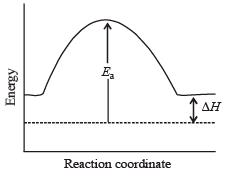Ea  >  ΔH

QUESTION: 5

During the kinetic study of the reaction, 2A + B → C + D, following results were obtained: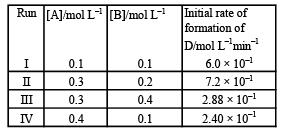Based on the above data which one of the following is correct? 

Solution:

In case of (II) and (III) Keeping concentration of [A] constant, when the concentration of [B] is doubled, the rate quadruples. Hence it is second order with respect to B. In case of I & IV Keeping the concentration of [B] constant. when the concentration of [A] is increased four times, rate also increases four times. Hence, the order with respect to A is one. hence Rate = k [A][B]2

QUESTION: 6

The rate of the reaction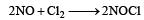is given by the rate equation rate = k [NO]2 [Cl2]      The value of the rate constant can be increased by:

Solution: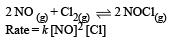The value of rate constant can be increased by increasing the temperature.
∴ Correct choice : (b)

QUESTION: 7

Which one of the following statements for the order of a reaction is incorrect ? 

Solution:

order of reaction may be zero, whole number or fractional.

QUESTION: 8

For the reaction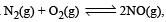, the equilibrium constant is K1. The equilibrium constant is K2 for the reaction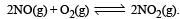.What is K for the reaction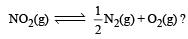Solution:

For the reaction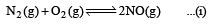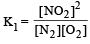For the reaction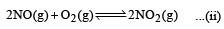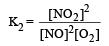For the reaction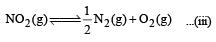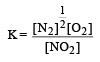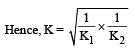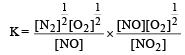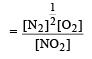QUESTION: 9

The rate of the reaction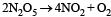can be written in three ways :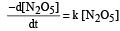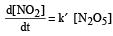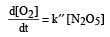The relationship between k and k' and between k and k"are :

[2011 M]

Solution:

Rate of disappearance of reactants = Rate of appearance of products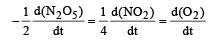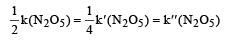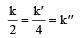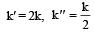QUESTION: 10

The unit of rate constant for a zero order reaction is [2011 M]

Solution:

Rate = K(A)0 Unit of K = mol L–1 sec–1

QUESTION: 11

In a reaction, A + B → Product, rate is doubled when the concentration of B is doubled, and rate increases by a factor of 8 when the concentrations of both the reactants (A and B) are doubled rate, law for the reaction can be written as : 

Solution:

According to statement given in the question, it is clear that r ∝ [A]2 and r ∝ [B]
That means order of reaction with respect to B is 1 and w.r.t A is 2
Hence, Rate = k[A]2[B]1

QUESTION: 12

In a zero-order reaction for every 10° rise of temperature, the rate is doubled. If the temperature is increased from 10°C to 100°C, the rate of the reaction will become : 

Solution: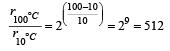*Multiple options can be correct
QUESTION: 13

Activation energy (Ea) and rate constants (k1 and k2) of a chemical reaction at two different temperatures (T1 and T2) are related by : [2012 M]

Solution:

According to Arrhenius equation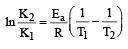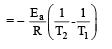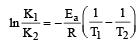QUESTION: 14

What is the activation energy for a reaction if its rate doubles when the temperature is raised from 20°C to 35°C? (R = 8.314 J mol–1 K–1) [NEET 2013]

Solution: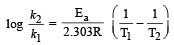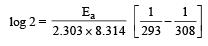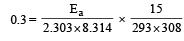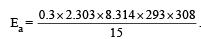= 34673 J mole-1
= 34.7 J mole-1

QUESTION: 15

A reaction having equal energies of activation for forward and reverse reaction has : [NEET 2013]

Solution: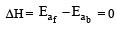QUESTION: 16

A reaction is 50% completed in 2 hours and 75% completed in 4 hours. The order of reaction is     [NEET Kar. 2013]

Solution:

For a first order reaction, t75% = 2 × t50%

QUESTION: 17

For a reaction between A and B the order with respect to A is 2 and the order with respect to B is 3. The concentrations of both A and B are doubled, the rate will increase by a factor of: [NEET Kar. 2013]

Solution:

Rate = k[A]2[B]3 when concentrations of both A and B are doubled then rate = k[2A]2[2B]3 = 32 k[A]2[B]3
∴       rate will increase by a factor of 32.